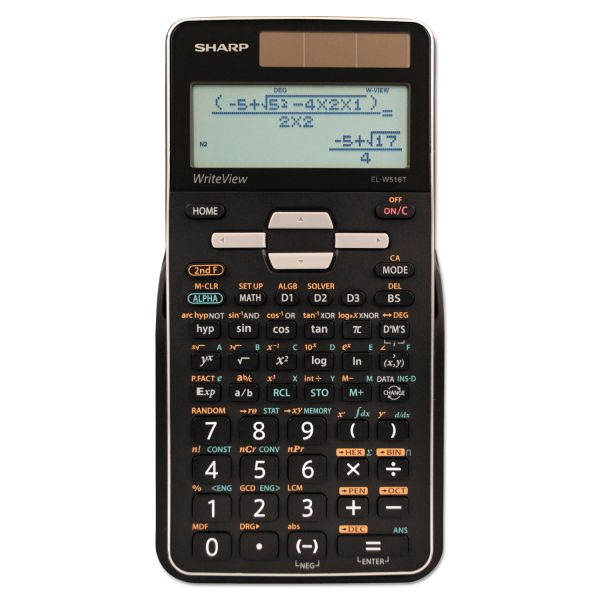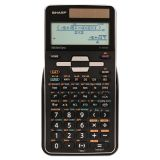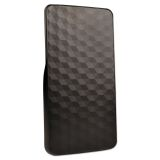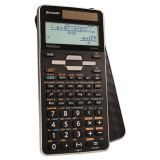Price Drop

# Sharp EL-W516TBSL Scientific Calculator, 16-Digit LCD

Product Number: SHRELW516TBSL
• WriteView Display.
• Large-16 digit, 4-line LCD display.
• 640 functions.
• Home key.
More details
\$24.66\$28.56
Free Shipping Orders Over \$45+ Estimated Delivery
Favorite
Compare
Request Quote

## Product Description

Sharp EL-W516TBSL Scientific Calculator, 16-Digit LCD - The EL-W516TB-SL model is perfect for students studying general math and science, pre-algebra, algebra, geometry, trigonometry, statistics, biology, and chemistry. This calculator performs 640 advanced scientific, math, and statistics functions. The EL-W516TB-SL utilizes a 4-line display featuring an exponent symbol. WriteView display allows users to view their input and results as they would appear in a textbook. The home key allows users to start fresh from any screen. This calculator has eight temporary memories, three definable memories, one independent memory, and one last answer memory. This calculator also allows equation editing & playback, 1 & 2 variable statistics, normal, stat, and drill modes, seven regression types, and N-BASE calculations: HEX, BIN, DEC, OCT, PEN. Other functions include: logarithms, reciprocals, powers, roots, factorials, trigs, and hyperbolic trigs. Includes a protective hard case and operates on twin-power (solar with battery backup).

• WriteView Display.
• Large-16 digit, 4-line LCD display.
• 640 functions.
• Home key.
• 7 Modes: Normal, Stat, Drill, Complex, Matrix, List & Equation.
• 8 temporary & 3 definable memory buttons.
• Quadratic & Cubic Equation Solvers.
• 3 Variable Linear Equation Solvers.
• 1 & 2 variable statistics.
• 3-D light reflecting hard cover.
• Twin-powered (solar with battery backup).Guides & Information

### Product Specifications

• +/- Switch Key
Yes
• Amortization
No
• Assembly Required
No
• Backspace Key
Yes
• Base Number Calculations
Yes
• Bond Calculations
No
• Brand Name
Sharp
• Case
Hard
• Cash Flow Calculations
No
• Complex Number Calculations
Yes
• Confidence Interval Calculating
No
• Cost/Sell/Margin
No
• Country of Origin
CN
• Currency Exchange Function
No
• Date Calculations
No
• Decimal Function
Yes
• Depreciation Calculations
No
• Display Angle
Fixed
• Display Characters Height
4 mm
• Display Characters x Display Lines
16 x 4
• Display Notation
Numeric
• Display Type(s)
LCD
• Display Window Resolution
96 x 32 Dot Matrix Display
• Double Zero Key
No
• Entry Logic
WriteView
• Equation Editor
Yes
• Fraction Calculations
Yes
• Fraction/Decimal Conversions
Yes
• Global Product Type
Calculators-Scientific
• Grand Total Key
No
• Graphing Functions
No
• Higher Mathematical Functions
Complex Drill Equation List Matrix Normal Statistics
• Hyperbolic Functions
Yes
• Hypothesis Testing
No
• I/O Port
No
• Interest Rate Conversion
No
• Item Count Function
No
• Levels of Parentheses
Unlimited
• Linear Regression
Yes
• Loan Calculation
No
• Logical (Boolean) Operations
Yes
• Markup/Down Key
No
• Matrices
Yes
• Memory
1 Independent 1 Last Answer 3 Definable 8 Temporary
• Metric Conversion
Yes
• Number of Display Digits
16
No
• Percent Key(s)
Yes
• Polar-Rectangular Conversion
Yes
• Post-Consumer Recycled Content Percent
0%
• Power Source(s)
Battery Solar
• Pre-Consumer Recycled Content Percent
0%
• Probability (Random Number)
Yes
• Programming Steps
No
• Recycled
No
• Replacement Batteries
LR44
• Simultaneous Equations
Yes
• Size
3.1 x 6.6
• Square Root Key
Yes
• Tax Calculation
No
• Time-Value-of-Money
No
• Time/Date
No
• Total Recycled Content Percent
0%
• Trig/Log Functions
Yes
• Variable Regression
Yes
• Variable Statistics
Yes
• Wall-Mountable
No